## Posts

### Concave, convex functions and points of inflection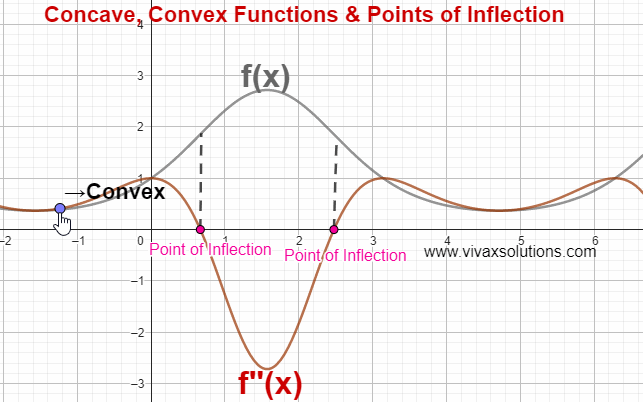Concave functions If f''(x) ≤ 0 in a given interval of x, the function is said to be concave. Convex functions If f''(x) ≥ 0 in a given interval of x, the function is said to be convex. Point of Inflection The point at which a curve changes being concave to convex or vice versa is called a point of inflection. E.g. f(x) = x 3 - 2x² + 5x - 4 f'(x) = 3x² - 4x + 5 f''(x) = 6x - 4 At the point of inflection, f''(x) = 0 6x - 4 = 0 x = 2/3 = 0.67 There is a point of inflection at x = 0.67. If x = 0.6 f''(0.6) = 3.6 - 4 = -0.4 f''(0.6) < 0 - the function is concave. If x = 0.8 f''(0.8) = 4.8 - 4 = 0.8 f''(0.8) > 0 - the function is convex. In the above simulation, there are two points of inflection. The simulation can be practised interactively here; just move the point gently to see the change.   Points of inflection on the Bell Curve - Normal Distribution There are two points of inflection on the bell curve.

### Happy New Year!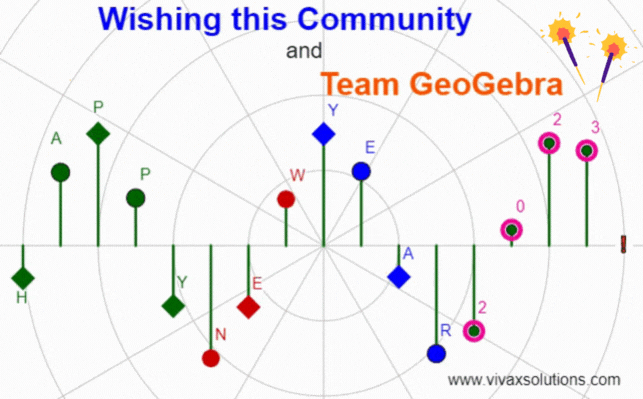### Differentiation: problem solving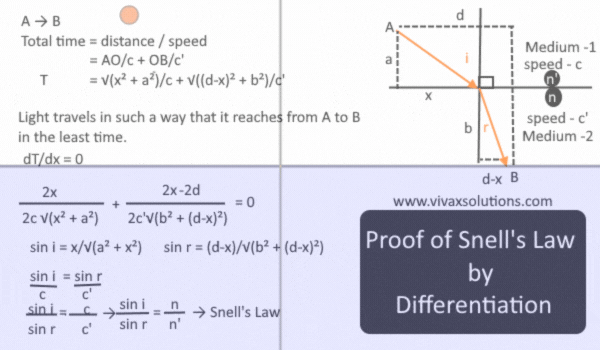Snell's Law When light refracts from one medium to another, the ratio of sine of the angles is equal to the ratio of refractive index of each corresponding medium. Snell's Law implies that light, when refracting from one medium to another, takes the shortest path. We can show by differentiation that when the light takes the shortest path, it obeys Snell's Law, verifying the 400-year-old law discovered  by the Dutch astronomer and mathematician.

### Pascal's Triangle in Python for Computer Science: GCSE, IGCSE and A Level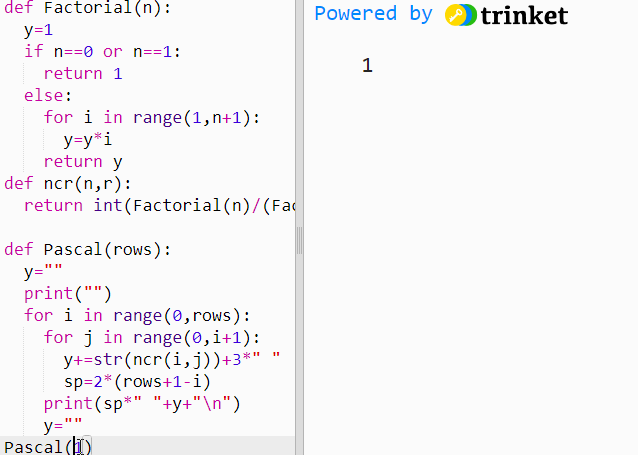The above simulation is produced in Python with aid of three functions. They are as follows: Factorial function  A function to generate combinations - nCr A function to produce the Pascal's Triangle You can practise it interactively with the following simulation. Please make sure you keep the number of rows below 10 so that the format of the output stays the way it should. The number of rows is the only parameter that Pascal(n) function needs.

### Straight Line Graph Generator for Maths Teachers: GCSE, IGCSE, GCE O Level, GCE A Level, A Level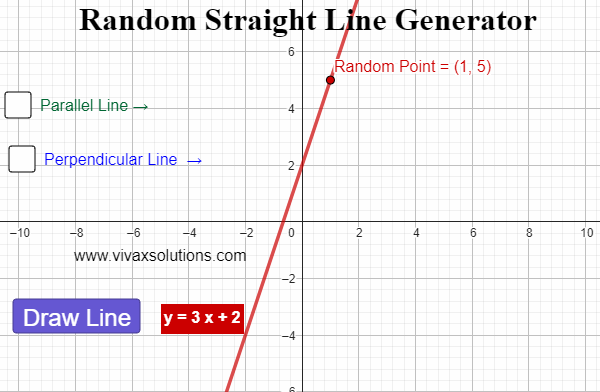Created with GeoGebra, this applet lets you generate straight line graphs at random, displaying the corresponding equation for each. In addition, you can find a parallel line - and its equation - for each line. Moreover, for each generated line, a random point is created. Then, the perpendicular line  that goes through the point in question can be created too. It is ideal for teachers at GCSE, IGCE, GCE O Level, GCE A Level and of course, A Level. The interactive simulation of the above is as follows: you can generate unlimited number of lines for practice for you students: just press the button - Draw Line -  to create random lines.

### Merry Christmas to all!Created with GeoGebra, the simulation shows how the potential energy of the Santa and the team, PE,  varies with the changing height of its their magical path to the ultimate destination.

### Creating Pascal's Triangle in C#: for computer science at GCSE, IGCE, A Level and programmers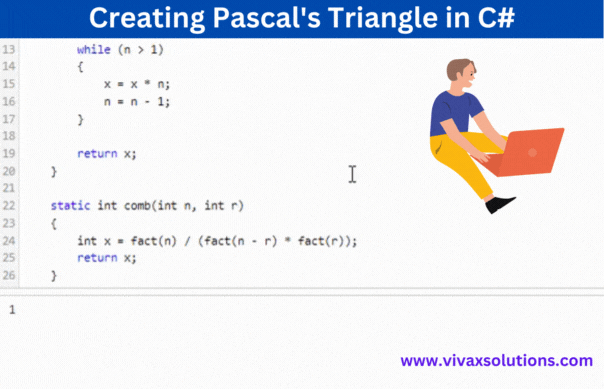Creation of Pascal's Triangle in C# The above animation is produced in C# programming language for students who do computer science at GCSE, IGCSE, IB and A Level. The code snippet consists of four major function that collectively produce the Pascal's Triangle. They are: A factorial function A function to generate combinations A function to generate Pascal's Triangle in the correct format The main function to run the function in step 3). The code snippet is given below; you can practise it interactively by providing the function, Pascal's Triangle(n) , the parameter required - the number of rows. Since the formatting is programmed up to 10 rows, please use a number below 10.